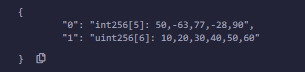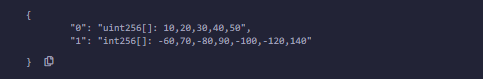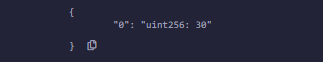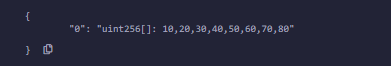# Solidity – Arrays

• Difficulty Level : Easy
• Last Updated : 11 May, 2022

Arrays are data structures that store the fixed collection of elements of the same data types in which each and every element has a specific location called index. Instead of creating numerous individual variables of the same type, we just declare one array of the required size and store the elements in the array and can be accessed using the index. In Solidity, an array can be of fixed size or dynamic size. Arrays have a continuous memory location, where the lowest index corresponds to the first element while the highest represents the last

### Creating an Array

To declare an array in Solidity, the data type of the elements and the number of elements should be specified. The size of the array must be a positive integer and data type should be a valid Solidity type

Syntax:

```<data type> <array name>[size] = <initialization>
```

### Fixed-size Arrays

The size of the array should be predefined. The total number of elements should not exceed the size of the array. If the size of the array is not specified then the array of enough size is created which is enough to hold the initialization.

Example: In the below example, the contract Types are created to demonstrate how to declare and initialize fixed-size arrays.

## Solidity

 `// Solidity program to demonstrate  ` `// creating a fixed-size array  ` `pragma solidity ^0.5.0;   ` ` `  `// Creating a contract  ` `contract` `Types {   ` ` `  `    ``// Declaring state variables ` `    ``// of type array ` `    ``uint data1;     ` `     `  `    ``// Defining function to add  ` `    ``// values to an array  ` `    ``function array_example() ``public` `returns ( ` `    ``int`` memory, uint memory){   ` `           `  `        ``int`` memory data  ` `        ``= [``int``(50), -63, 77, -28, 90];   ` `        ``data1  ` `        ``= [uint(10), 20, 30, 40, 50, 60]; ` `           `  `        ``return` `(data, data1);   ` `  ``}   ` `}`

Output :### Dynamic Array:

The size of the array is not predefined when it is declared. As the elements are added the size of array changes and at the runtime, the size of the array will be determined.

Example: In the below example, the contract Types are created to demonstrate how to create and initialize dynamic arrays.

## Solidity

 `// Solidity program to demonstrate  ` `// creating a dynamic array ` `pragma solidity ^0.5.0;   ` ` `  `// Creating a contract   ` `contract` `Types {   ` `   `  `    ``// Declaring state variable  ` `    ``// of type array. One is fixed-size ` `    ``// and the other is dynamic array ` `    ``uint[] data  ` `      ``= [10, 20, 30, 40, 50];  ` `    ``int``[] data1;   ` `   `  `    ``// Defining function to  ` `    ``// assign values to dynamic array ` `    ``function dynamic_array() ``public` `returns( ` `      ``uint[] memory, ``int``[] memory){   ` `   `  `        ``data1  ` `          ``= [``int``(-60), 70, -80, 90, -100, -120, 140];  ` `        ``return` `(data, data1);   ` `    ``}   ` `}`

Output :### Array Operations

1. Accessing Array Elements: The elements of the array are accessed by using the index. If you want to access ith element then you have to access (i-1)th index.

Example: In the below example, the contract Types first initializes an array[data] and then retrieves the value at specific index 2.

## Solidity

 `// Solidity program to demonstrate ` `// accessing elements of an array ` `pragma solidity ^0.5.0;   ` `  `  `// Creating a contract  ` `contract` `Types {   ` ` `  `    ``// Declaring an array ` `    ``uint data;     ` `      `  `    ``// Defining function to  ` `    ``// assign values to array ` `    ``function array_example( ` `    ``) ``public` `payable returns (uint memory){   ` `           `  `        ``data  ` `          ``= [uint(10), 20, 30, 40, 50, 60]; ` `        ``return` `data;   ` `  ``}  ` `   `  `  ``// Defining function to access ` `  ``// values from the array ` `  ``// from a specific index   ` `  ``function array_element( ` `  ``) ``public` `payable returns (uint){   ` `        ``uint x = data; ` `        ``return` `x;   ` `  ``}   ` `}`

Output :2. Length of Array: Length of the array is used to check the number of elements present in an array. The size of the memory array is fixed when they are declared, while in case the dynamic array is defined at runtime so for manipulation length is required.

Example: In the below example, the contract Types first initializes an array[data] and then the length of the array is calculated.

## Solidity

 `// Solidity program to demonstrate  ` `// how to find length of an array ` `pragma solidity ^0.5.0;   ` ` `  `// Creating a contract ` `contract` `Types {   ` ` `  `    ``// Declaring an array ` `    ``uint data;     ` `       `  `    ``// Defining a function to  ` `    ``// assign values to an array ` `    ``function array_example( ` `    ``) ``public` `payable returns (uint memory){   ` `        ``data = [uint(10), 20, 30, 40, 50, 60]; ` `        ``return` `data;   ` `  ``}   ` ` `  `  ``// Defining a function to  ` `  ``// find the length of the array ` `  ``function array_length( ` `  ``) ``public` `returns(uint) {   ` `        ``uint x = data.length; ` `        ``return` `x;  ` `    ``}  ` `  ``}`

Output :3. Push: Push is used when a new element is to be added in a dynamic array. The new element is always added at the last position of the array.

Example: In the below example, the contract Types first initializes an array[data], and then more values are pushed into the array.

## Solidity

 `// Solidity program to demonstrate  ` `// Push operation ` `pragma solidity ^0.5.0;   ` `  `  `// Creating a contract  ` `contract` `Types {   ` ` `  `    ``// Defining the array ` `    ``uint[] data = [10, 20, 30, 40, 50];  ` `   `  `    ``// Defining the function to push  ` `    ``// values to the array ` `    ``function array_push( ` `    ``) ``public` `returns(uint[] memory){   ` `   `  `        ``data.push(60);   ` `        ``data.push(70);   ` `        ``data.push(80); ` `   `  `        ``return` `data;   ` `    ``}   ` `}`

Output :4. Pop: Pop is used when the last element of the array is to be removed in any dynamic array.

Example: In the below example, the contract Types first initializes an array[data], and then values are removed from the array using the pop function.

## Solidity

 `// Solidity program to demonstrate ` `// Pop operation ` `pragma solidity ^0.5.0;   ` `   `  `// Creating a contract ` `contract` `Types {   ` ` `  `    ``// Defining an array ` `    ``uint[] data  ` `      ``= [10, 20, 30, 40, 50]; ` `   `  `    ``// Defining a function to  ` `    ``// pop values from the array ` `    ``function array_pop( ` `    ``) ``public` `returns(uint[] memory){   ` `        ``data.pop();  ` `        ``return` `data;   ` `    ``}   ` `}`

Output :My Personal Notes arrow_drop_up
Recommended Articles
Page :